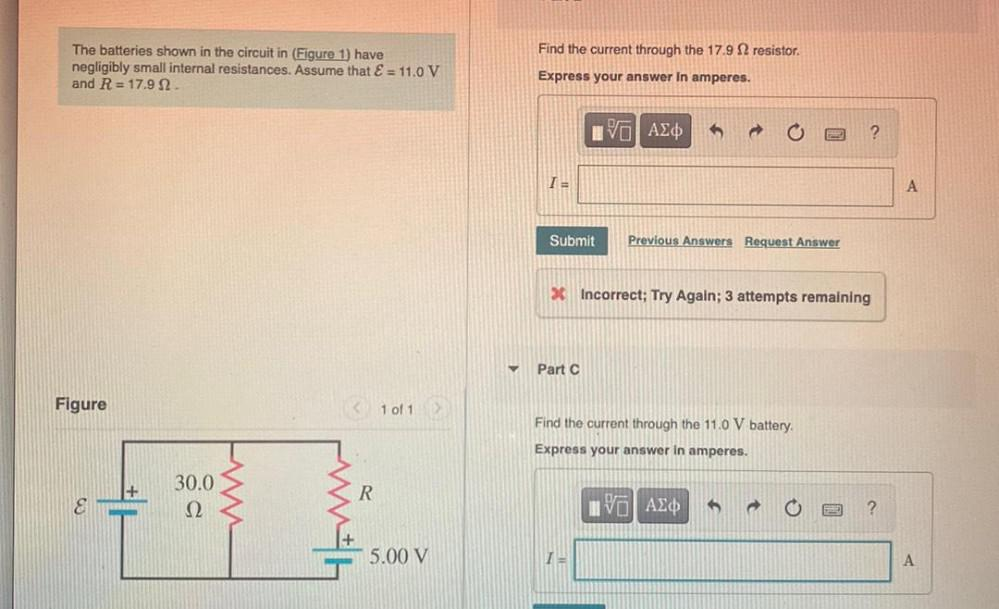Question:

# Find the current through the 17.9 12 resistor. The batteries shown in the circuit in (Figure 1) have negligibly small internal rFind the current through the 17.9 12 resistor. The batteries shown in the circuit in (Figure 1) have negligibly small internal resistances. Assume that E = 11.0 V and R = 17.9 12 Express your answer In amperes. IVO ALO ? TE A Submit Previous Answers Request Answer * Incorrect; Try Again; 3 attempts remaining Part C Figure < 1 of 1 Find the current through the 11.0 V battery. Express your answer in amperes. 30.0 22 R IVO AE ? W with 5.00 V I A